# Programming Using Python>>>>>>>>>>>>>>>>>>>>>>>> (Algebra: linear equations) Design a class named LinearEquation for 2x2 a system of...

Programming Using Python>>>>>>>>>>>>>>>>>>>>>>>>

(Algebra: linear equations) Design a class named LinearEquation for 2x2 a system of linear equations: The class contains:

? The private data fields a, b, c, d, e, and f with get methods.

? A constructor with the arguments for a, b, c, d, e, and f.

? Six get methods for a, b, c, d, e, and f.

? A method named isSolvable() that returns true if is not 0.

? The methods getX() and getY() that return the solution for the equation.

Draw the UML diagram for the class, and then implement the class. Write a test program that prompts the user to enter a, b, c, d, e, and f and displays the result. If ad – bc is 0, or report that “The equation has no solution.” The Rectangle class, design a class named Rectangle to represent a rectangle.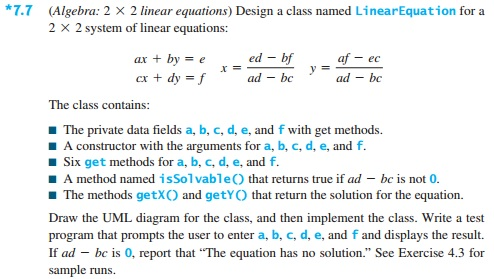This Homework Help Question: "Programming Using Python>>>>>>>>>>>>>>>>>>>>>>>> (Algebra: linear equations) Design a class named LinearEquation for 2x2 a system of..." No answers yet.

We need 10 more requests to produce the answer to this homework help question. Share with your friends to get the answer faster!

0 /10 have requested the answer to this homework help question.

Once 10 people have made a request, the answer to this question will be available in 1-2 days.
All students who have requested the answer will be notified once they are available.

#### Earn Coin

Coins can be redeemed for fabulous gifts.

Similar Homework Help Questions
• ### java code Design a class named QuadraticEquation for a quadratic equation with real coefficients ax? +...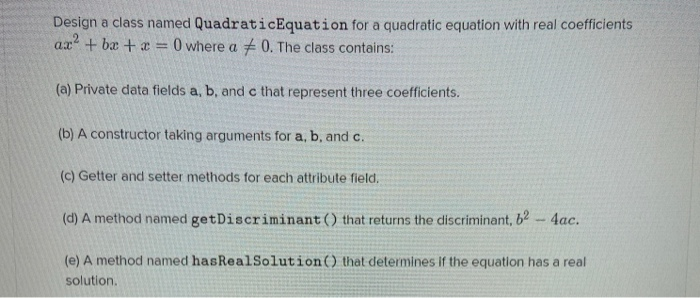java code Design a class named QuadraticEquation for a quadratic equation with real coefficients ax? + bx + x = 0 where a = 0. The class contains: (a) Private data fields a, b, and c that represent three coefficients. (b) A constructor taking arguments for a, b, and c. (c) Getter and setter methods for each attribute field. (d) A method named getDiscriminant() that returns the discriminant, 62 - 4ac. (e) A method named hasReal Solution that determines If...

• ### C++ Java - Exercise: Design a class named Rectangle that represents a rectangle. The class...

C++ programming class. Please make as easy to understand as possible as this is just a beginning course. Thanks!Design a class named Rectangle that represents a rectangle. The class should contain the following:• Two private double data fields named “width” and “height” where the default width and height values are 1• A no-arg constructor that creates a default rectangle• A constructor that creates a rectangle with the specified width and height• A method named getArea() that returns the area of...

• ### Java Programming Design a class named Person and its two subclasses named Student and Employee. A...

Java Programming Design a class named Person and its two subclasses named Student and Employee. A person has a name, address, phone number, and email address. A student has a class status (freshman, sophomore, junior, or senior). Define the status as a constant. An employee has an office, salary, date hired. Define a class named MyDate that contains the fields year, month, and day. Override the toString method in each class to display the class name and the person's name....

Design a class named QuadraticEquation for a quadratic equation ax2+bx+x=0. The class contains:private data fields a, b, and c that represents three coefficients.a constructor for the arguments for a, b, and c.three get methods for a, b, and c.a method named getDiscriminant() that returns the discriminant, which is b2-4acthe methods named getRoot1() and getRoot2() for returning two roots of the equation, or returning 0 if the discriminant is negative.Write a test program that prompts the user to enter values for...

• ### It's been too long since I have done any Java programming. Can anyone help me with...

It's been too long since I have done any Java programming. Can anyone help me with this one? Thank you 6.5 Write the declaration for a class named C that declares: (1) a private int instance variable named mX; (2) a private int class variable named mY initialized to 0; (3) a private int class constant named A which is equivalent to 100; (4) a public int class constant named B which is equivalent to 200; (5) public accessor and...

• ### PYTHON PROGRAMMING LANGUAGE Design a class named Savings Account that contains: A private int data field...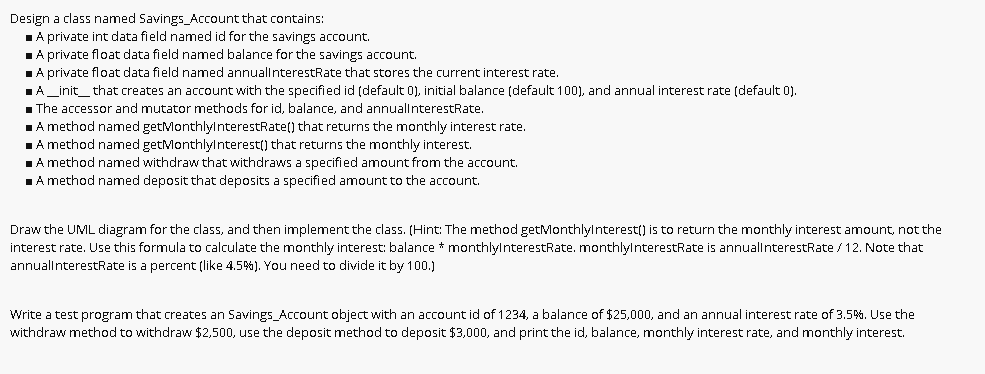PYTHON PROGRAMMING LANGUAGE Design a class named Savings Account that contains: A private int data field named id for the savings account. A private float data field named balance for the savings account. A private float data field named annuallnterestRate that stores the current interest rate. A_init_ that creates an account with the specified id (default 0), initial balance (default 100), and annual interest rate (default 0). . The accessor and mutator methods for id, balance, and annuallnterestRate. A method...

• ### java language Problem 3: Shape (10 points) (Software Design) Create an abstract class that represents a Shape and contains abstract method: area and perimeter. Create concrete classes: Circle, Rectang...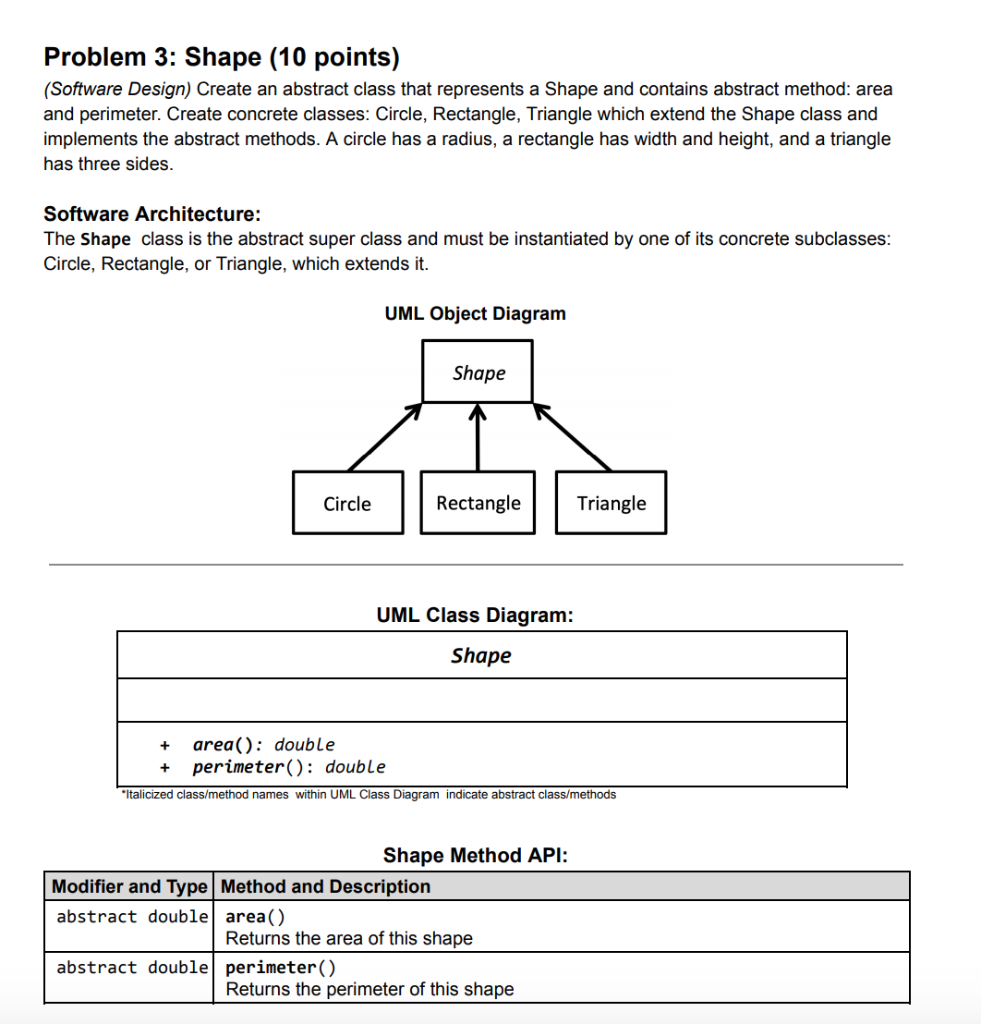java language Problem 3: Shape (10 points) (Software Design) Create an abstract class that represents a Shape and contains abstract method: area and perimeter. Create concrete classes: Circle, Rectangle, Triangle which extend the Shape class and implements the abstract methods. A circle has a radius, a rectangle has width and height, and a triangle has three sides. Software Architecture: The Shape class is the abstract super class and must be instantiated by one of its concrete subclasses: Circle, Rectangle, or...

• ### Linear Algebra 1) For each of the following linear systems of equations I. 2x, x 3...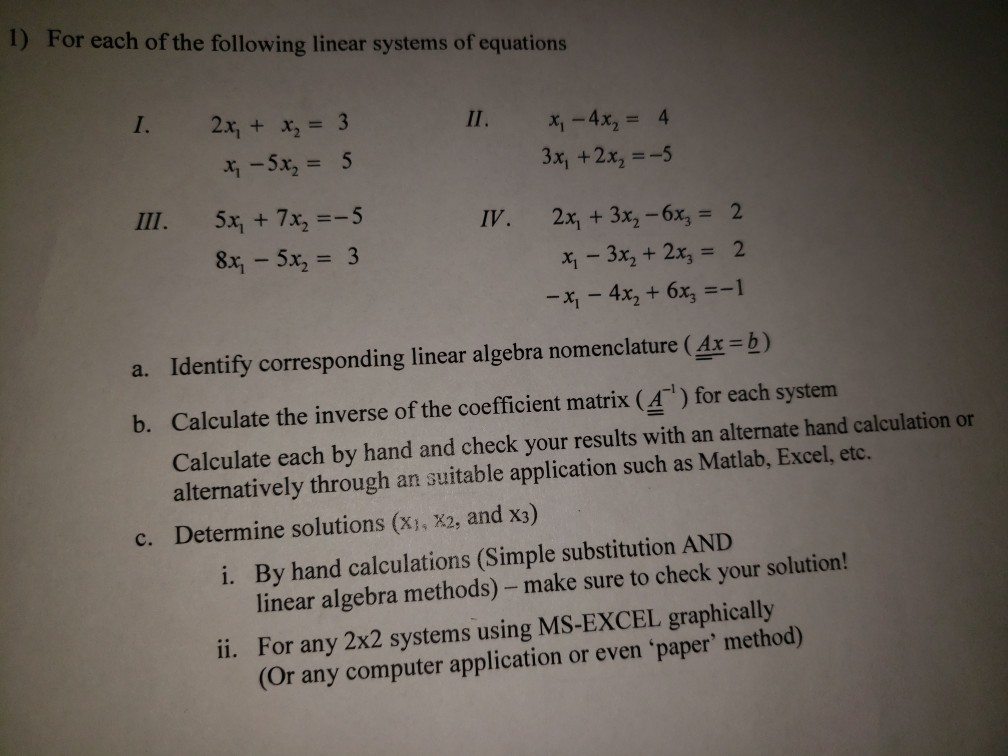Linear Algebra 1) For each of the following linear systems of equations I. 2x, x 3 x,-4x2 = 4 3x, +2x-5 2x, + 3x2-6x3 x 3x2 + 2x 2 -x,-4x2 + 6x3 =-1 III. 5x1 + 7x2=-5 8x1-5x2 = 3 IV, 2 a. Identify corresponding linear algebra nomenclature (4x -b) b. Calculate the inverse of the coefficient matrix (4) for each system Calculate each by hand and check your results with an alternate hand calculation or alternatively through an suitable...

• ### java language please. thank you T-Mobile 9:00 AM For this assignment, create a Java class named...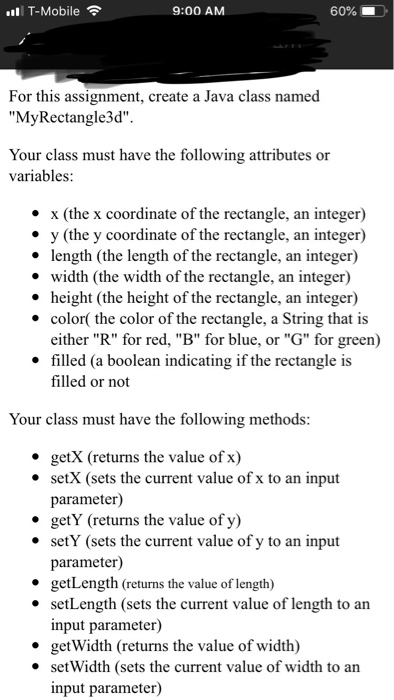java language please. thank you T-Mobile 9:00 AM For this assignment, create a Java class named "MyRectangle3d" Your class must have the following attributes or variables · x (the x coordinate of the rectangle, an integer) * y (the y coordinate of the rectangle, an integer) * length (the length of the rectangle, an integer) * width (the width of the rectangle, an integer) * height (the height of the rectangle, an integer) * color( the color of the rectangle,...

• ### I am just starting out in programming

I am just starting out in programming. If someone could help me with these assignments, it would be greatly appreciated. "Write a Rectangle class. This class represents Rectangles. Each Rectange has the X and Y coordinates of its center point (as doubles) and its width and height (as double values, too). No other values should be stored! This means no area, no perimeter! The class should have the following constructors and methods: 1. a constructor that takes X, Y, width,...